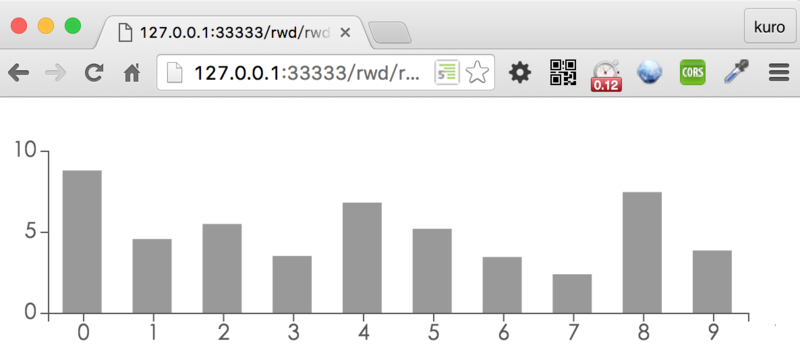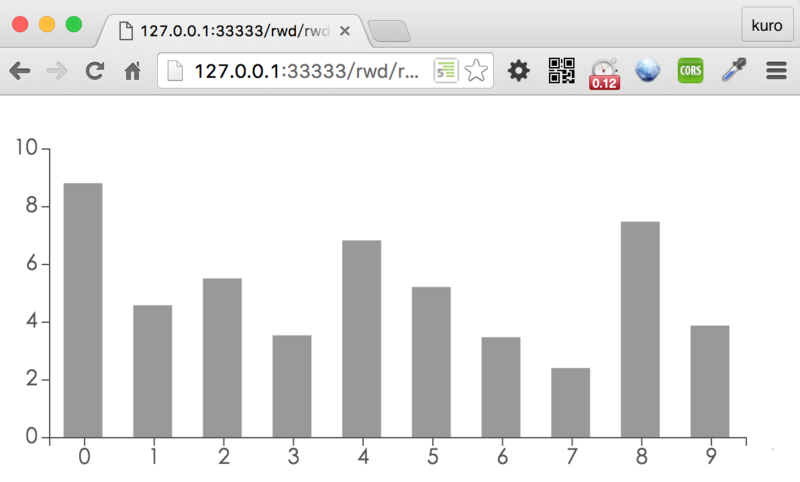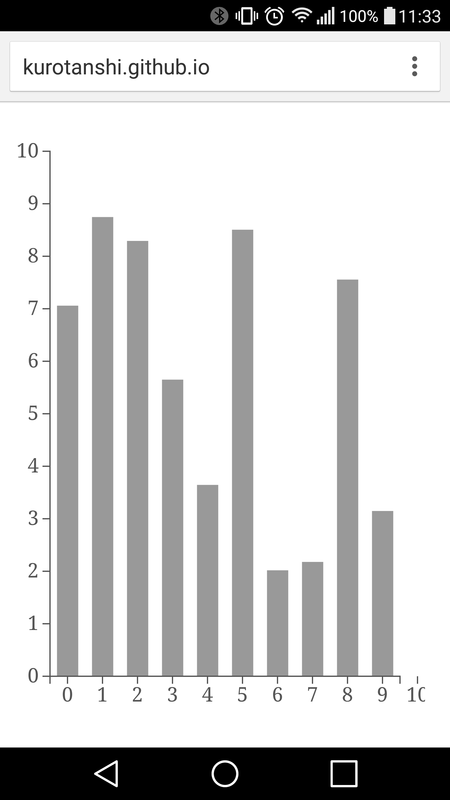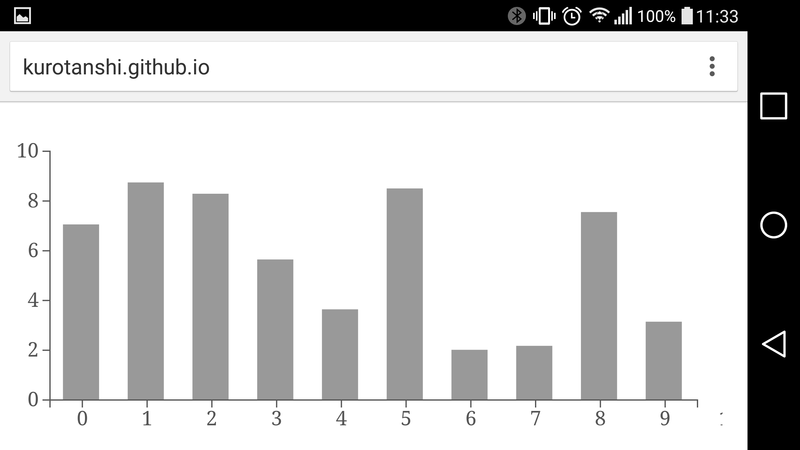[Code]

``````  var margin = 40,
width = 960 - margin*2,
height = 500 - margin*2;
``````

``````.content{
display: block;
width: 100%;
height: 100%;
min-width: 300px;
max-width: 960px;
max-height: 500px;
overflow: hidden;
}
``````
`````` // 將尺寸改成即時取得的寬高
width = parseInt(d3.select(".content").style("width"), 10) - margin*2;
height = parseInt(d3.select(".content").style("height"), 10) - margin*2;
``````

``````  var svg = d3.select('.svg');

// 將繪製動作包裝至 function 內
function rendering() {
// 將繪製的程式碼通通搬到裡面
// 內略
}

// 將 window 綁定 resize 事件，並重新繪製圖型
d3.select(window).on('resize', rendering);

// 首次繪製
rendering();
``````

[Code] 都是沒有問題的。

``````  // y 軸加上 ticks
var yAxis = d3.svg.axis()
.scale(yScale2)
.orient("left")
.ticks(Math.max(height/50, 2));
``````

`Math.max` 會回傳兩個指定數字中較大的一個，而 `ticks()` 則是設定軸線上刻度的數量。[Code]